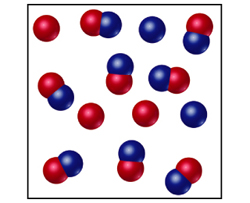# Problem: The following diagram represents an equilibrium mixture produced for a reaction of the type A + X .Is K greater or smaller than 1 if the volume is 1 L and each atom/molecule in the diagram represents 1 mol?

🤓 Based on our data, we think this question is relevant for Professor Hurst's class at LSU.

###### FREE Expert Solution

Recall that if K > 1, full dissociation is expected

For K < 1, partial dissociation is observed###### Problem Details

The following diagram represents an equilibrium mixture produced for a reaction of the type A + X .Is K greater or smaller than 1 if the volume is 1 L and each atom/molecule in the diagram represents 1 mol?